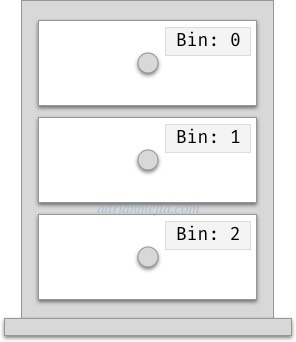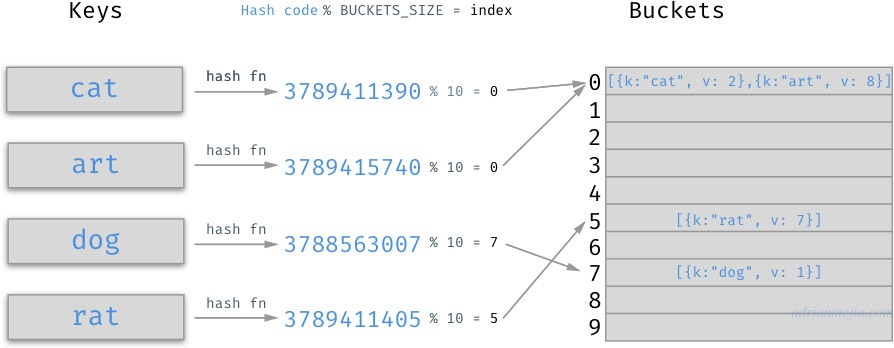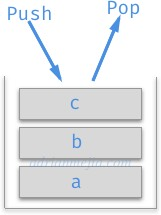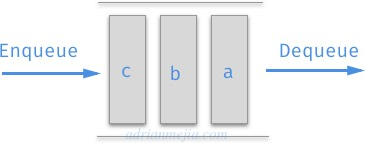sea_ljf

## 初学者应该了解的数据结构：Array、HashMap 与 List1. 算法的时间复杂性与大 O 符号
2. 每个程序员应该知道的八种时间复杂度
3. 初学者应该了解的数据结构：Array、HashMap 与 List 👈 即本文
4. 初学者应该了解的数据结构： Graph
5. 初学者应该了解的数据结构：Tree (敬请期待)
6. 附录 I：递归算法分析

# （操作）数据结构的时间复杂度

* = 运行时分摊

Array O(n) O(1) O(n) O(n) 插入最后位置复杂度为 O(1)
(Hash)Map O(1)* O(1)* O(1)* O(1)* 重新计算哈希会影响插入时间。
Map O(log(n)) - O(log(n)) O(log(n)) 通过二叉搜索树实现
Set（使用 HashMap） O(1）* - O(1)* O(1)* 由 HashMap 实现
Set (使用 List) O(n) - O(n)] O(n) 通过 List 实现
Set (使用二叉搜索树) O(log(n)) - O(log(n)) O(log(n)) 通过二叉搜索树实现
Linked List (单向) O(n) - O(n) O(n) 在起始位置添加或删除元素，复杂度为 O(1)
Linked List (双向） O(n) - O(n) O(n) 在起始或结尾添加或删除元素，复杂度为 O(1)。然而在其他位置是 O(n)
Stack (由 Array 实现) O(1) - - O(1)] 插入与删除都遵循与后进先出（LIFO）
Queue (简单地由 Array 实现) O(n) - - O(1) 插入（Array.shift）操作的复杂度是 O(n)
Queue (由 Array 实现，但进行了改进) O(1)* - - O(1) 插入操作的最差情况复杂度是 O(n)。然而分摊后是 O(1)
Queue (由 List 实现) O(1) - - O(1) 使用双向链表

# 原始数据类型

• 整数，如：1, 2, 3, …
• 字符，如：a, b, "1", "*"
• 布尔值， true 与 false.
• 浮点数 ，如：3.14159, 1483e-2.

# Array## Array 的内置方法

array.push(element1[, …[, elementN]]) O(1) 将一个或多个元素添加到数组的末尾
array.pop() O(1) 移除数组末尾的元素
array.shift() O(n) 移除数组开头的元素
array.unshift(element1[, …[, elementN]]) O(n) 将一个或多个元素添加到数组的开头
array.slice([beginning[, end]]) O(n) 返回浅拷贝原数组从 `beginning``end`（不包括 `end`）部分组成的新数组
array.splice(start[, deleteCount[, item1[,…]]]) O(n) 改变 (插入或删除) 数组

## 向数组插入元素

``````function insertToTail(array, element) {
array.push(element);
return array;
}

const array = [1, 2, 3];
console.log(insertToTail(array, 4)); // => [ 1, 2, 3, 4 ]
``````

`Array.push` 的时间复杂度度是 O(1)

``````function insertToHead(array, element) {
array.unshift(element);
return array;
}

const array = [1, 2, 3];
console.log(insertToHead(array, 0));// => [ 0, 1, 2, 3, ]
``````

`Array.unshift` 的时间复杂度度是 O(n)

## 访问数组中的元素

``````function access(array, index) {
return array[index];
}

const array = [1, 'word', 3.14, { a: 1 }];
access(array, 0);// => 1
access(array, 3);// => {a: 1}
``````

## 在数组中查找元素

``````function search(array, element) {
for (let index = 0;
index < array.length;
index++) {
if (element === array[index]) {
return index;
}
}
}

const array = [1, 'word', 3.14, { a: 1 }];
console.log(search(array, 'word'));// => 1
console.log(search(array, 3.14));// => 2
``````

## 在数组中删除元素

1. 从数组的末尾删除元素所需时间是恒定的，也就是 O(1)
2. 然而，无论是从数组的开头或是中间位置删除元素，你都需要调整（删除元素后面的）元素位置。因此复杂度为 O(n)

``````function remove(array, element) {
const index = search(array, element);
array.splice(index, 1);
return array;
}

const array1 = [0, 1, 2, 3];
console.log(remove(array1, 1));// => [ 0, 2, 3 ]
``````

# HashMaps

HashMap有很多名字，如 HashTableHashMap、Map、Dictionary、Associative Array 等。概念上它们都是一致的，实现上稍有不同。

HashMap 也和抽屉一样存储东西，通过不同标识来区分不同匣子。1. 数组：通过哈希函数将键映射为数组的索引。（查找）最差情况： O(n)，平均： O(1)。
2. 二叉搜索树: 使用自平衡二叉搜索树查找值（另外的文章会详细介绍）。 （查找）最差情况： O(log n)，平均：O(log n)## HashMap 的简单实现

``````class NaiveHashMap {

constructor(initialCapacity = 2) {
this.buckets = new Array(initialCapacity);
}

set(key, value) {
const index = this.getIndex(key);
this.buckets[index] = value;
}

get(key) {
const index = this.getIndex(key);
return this.buckets[index];
}

hash(key) {
return key.toString().length;
}

getIndex(key) {
const indexHash = this.hash(key);
const index = indexHash % this.buckets.length;
return index;
}
}
``````

HashMap 的初始容量（译者注：容量指的是用于存储数据的数组长度，即桶的数量）是2（两个桶）。当我们往里面存储多个元素时，通过求余 `%` 计算出该键应存入桶的编号（，并将数据存入该桶中）。

``````// Usage:
const assert = require('assert');
const hashMap = new NaiveHashMap();
hashMap.set('cat', 2);
hashMap.set('rat', 7);
hashMap.set('dog', 1);
hashMap.set('art', 8);
console.log(hashMap.buckets);
/*
bucket #0: <1 empty item>,
bucket #1: 8
*/
assert.equal(hashMap.get('art'), 8); // this one is ok
assert.equal(hashMap.get('cat'), 8); // got overwritten by art 😱
assert.equal(hashMap.get('rat'), 8); // got overwritten by art 😱
assert.equal(hashMap.get('dog'), 8); // got overwritten by art 😱
``````

1) Hash function 转换出太多相同的索引。如：

``````hash('cat') // 3
hash('dog') // 3
``````

2) 完全不处理冲突的情况。`cat``dog` 会重写彼此在 HashMap 中的值（它们均在桶 #1 中）。

3 数组长度。 即使我们有一个更好的哈希函数，由于数组的长度是2，少于存入元素的数量，还是会产生很多冲突。我们希望 HashMap 的初始容量大于我们存入数据的数量。

## 改进哈希函数

HashMap 的主要目标是将数组查找与访问的时间复杂度，从 O(n) 降至 O(1)

1. 一个合适的哈希函数，尽可能地减少冲突。
2. 一个长度足够大的数组用于保存数据。

``````hash(key) {
let hashValue = 0;
const stringKey = key.toString();
for (let index = 0; index < stringKey.length; index++) {
const charCode = stringKey.charCodeAt(index);
hashValue += charCode;
}
return hashValue;
}
``````

``````hash('cat') // 312  (c=99 + a=97 + t=116)
hash('dog') // 314 (d=100 + o=111 + g=103)
``````

``````hash(key) {
let hashValue = 0;
const stringKey = `\${key}`;
for (let index = 0; index < stringKey.length; index++) {
const charCode = stringKey.charCodeAt(index);
hashValue += charCode << (index * 8);
}
return hashValue;
}
``````

``````// r = 114 or 0x72; a = 97 or 0x61; t = 116 or 0x74

hash('rat'); // 7,627,122 (r: 114 * 1 + a: 97 * 256 + t: 116 * 65,536) or in hex: 0x726174 (r: 0x72 + a: 0x6100 + t: 0x740000)

hash('art'); // 7,631,457 or 0x617274
``````

``````hash(1); // 49
hash('1'); // 49
hash('1,2,3'); // 741485668
hash([1,2,3]); // 741485668
hash('undefined') // 3402815551
hash(undefined) // 3402815551
``````

``````hash(key) {
let hashValue = 0;
const stringTypeKey = `\${key}\${typeof key}`;
for (let index = 0; index < stringTypeKey.length; index++) {
const charCode = stringTypeKey.charCodeAt(index);
hashValue += charCode << (index * 8);
}
return hashValue;
}
``````

``````console.log(hash(1)); // 1843909523
console.log(hash('1')); // 1927012762
console.log(hash('1,2,3')); // 2668498381
console.log(hash([1,2,3])); // 2533949129
console.log(hash('undefined')); // 5329828264
console.log(hash(undefined)); // 6940203017
``````

Yay!!! 🎉 我们终于有了更好的哈希函数！

## 更完善的 HashMap 实现

• 哈希函数， 检查类型与计算各字符（ascii 码的总和）以减少冲突的发生。
• 处理冲突，通过将值添加到集合中来解决这问题，同时需要一个计数器追踪冲突的数量。

``````class DecentHashMap {
constructor(initialCapacity = 2) {
this.buckets = new Array(initialCapacity);
this.collisions = 0;
}
set(key, value) {
const bucketIndex = this.getIndex(key);
if(this.buckets[bucketIndex]) {
this.buckets[bucketIndex].push({key, value});
if(this.buckets[bucketIndex].length > 1) { this.collisions++; }
} else {
this.buckets[bucketIndex] = [{key, value}];
}
return this;
}
get(key) {
const bucketIndex = this.getIndex(key);
for (let arrayIndex = 0; arrayIndex < this.buckets[bucketIndex].length; arrayIndex++) {
const entry = this.buckets[bucketIndex][arrayIndex];
if(entry.key === key) {
return entry.value
}
}
}
hash(key) {
let hashValue = 0;
const stringTypeKey = `\${key}\${typeof key}`;
for (let index = 0; index < stringTypeKey.length; index++) {
const charCode = stringTypeKey.charCodeAt(index);
hashValue += charCode << (index * 8);
}
return hashValue;
}
getIndex(key) {
const indexHash = this.hash(key);
const index = indexHash % this.buckets.length;
return index;
}
}
``````

``````// Usage:
const assert = require('assert');
const hashMap = new DecentHashMap();
hashMap.set('cat', 2);
hashMap.set('rat', 7);
hashMap.set('dog', 1);
hashMap.set('art', 8);
console.log('collisions: ', hashMap.collisions); // 2
console.log(hashMap.buckets);
/*
bucket #0: [ { key: 'cat', value: 2 }, { key: 'art', value: 8 } ]
bucket #1: [ { key: 'rat', value: 7 }, { key: 'dog', value: 1 } ]
*/
assert.equal(hashMap.get('art'), 8); // this one is ok
assert.equal(hashMap.get('cat'), 2); // Good. Didn't got overwritten by art
assert.equal(hashMap.get('rat'), 7); // Good. Didn't got overwritten by art
assert.equal(hashMap.get('dog'), 1); // Good. Didn't got overwritten by art
``````

``````hash('cat') => 3789411390; bucketIndex => 3789411390 % 2 = 0
hash('art') => 3789415740; bucketIndex => 3789415740 % 2 = 0
hash('dog') => 3788563007; bucketIndex => 3788563007 % 2 = 1
hash('rat') => 3789411405; bucketIndex => 3789411405 % 2 = 1
``````

``````const hashMapSize10 = new DecentHashMap(10);
hashMapSize10.set('cat', 2);
hashMapSize10.set('rat', 7);
hashMapSize10.set('dog', 1);
hashMapSize10.set('art', 8);
console.log('collisions: ', hashMapSize10.collisions); // 1
console.log('hashMapSize10\n', hashMapSize10.buckets);
/*
bucket#0: [ { key: 'cat', value: 2 }, { key: 'art', value: 8 } ],
<4 empty items>,
bucket#5: [ { key: 'rat', value: 7 } ],
<1 empty item>,
bucket#7: [ { key: 'dog', value: 1 } ],
<2 empty items>
*/
````````````const hashMapSize100 = new DecentHashMap(100);
hashMapSize100.set('cat', 2);
hashMapSize100.set('rat', 7);
hashMapSize100.set('dog', 1);
hashMapSize100.set('art', 8);
console.log('collisions: ', hashMapSize100.collisions); // 0
console.log('hashMapSize100\n', hashMapSize100.buckets);
/*
<5 empty items>,
bucket#5: [ { key: 'rat', value: 7 } ],
<1 empty item>,
bucket#7: [ { key: 'dog', value: 1 } ],
<32 empty items>,
bucket#41: [ { key: 'art', value: 8 } ],
<49 empty items>,
bucket#90: [ { key: 'cat', value: 2 } ],
<9 empty items>
*/
``````

Yay! 🎊 没有冲突！

## 优化HashMap 的实现

（从开始就）分配大量的内存（去建立数组）是不合理的。因此，我们能做的是根据装载因子动态地调整容量。这操作被称为 rehash

``````class HashMap {
constructor(initialCapacity = 16, loadFactor = 0.75) {
this.buckets = new Array(initialCapacity);
this.size = 0;
this.collisions = 0;
this.keys = [];
}
hash(key) {
let hashValue = 0;
const stringTypeKey = `\${key}\${typeof key}`;
for (let index = 0; index < stringTypeKey.length; index++) {
const charCode = stringTypeKey.charCodeAt(index);
hashValue += charCode << (index * 8);
}
return hashValue;
}
_getBucketIndex(key) {
const hashValue = this.hash(key);
const bucketIndex = hashValue % this.buckets.length;
return bucketIndex;
}
set(key, value) {
const {bucketIndex, entryIndex} = this._getIndexes(key);
if(entryIndex === undefined) {
// initialize array and save key/value
const keyIndex = this.keys.push({content: key}) - 1; // keep track of the key index
this.buckets[bucketIndex] = this.buckets[bucketIndex] || [];
this.buckets[bucketIndex].push({key, value, keyIndex});
this.size++;
// Optional: keep count of collisions
if(this.buckets[bucketIndex].length > 1) { this.collisions++; }
} else {
// override existing value
this.buckets[bucketIndex][entryIndex].value = value;
}
// check if a rehash is due
this.rehash(this.buckets.length * 2);
}
return this;
}
get(key) {
const {bucketIndex, entryIndex} = this._getIndexes(key);
if(entryIndex === undefined) {
return;
}
return this.buckets[bucketIndex][entryIndex].value;
}
has(key) {
return !!this.get(key);
}
_getIndexes(key) {
const bucketIndex = this._getBucketIndex(key);
const values = this.buckets[bucketIndex] || [];
for (let entryIndex = 0; entryIndex < values.length; entryIndex++) {
const entry = values[entryIndex];
if(entry.key === key) {
return {bucketIndex, entryIndex};
}
}
return {bucketIndex};
}
delete(key) {
const {bucketIndex, entryIndex, keyIndex} = this._getIndexes(key);
if(entryIndex === undefined) {
return false;
}
this.buckets[bucketIndex].splice(entryIndex, 1);
delete this.keys[keyIndex];
this.size--;
return true;
}
rehash(newCapacity) {
const newMap = new HashMap(newCapacity);
this.keys.forEach(key => {
if(key) {
newMap.set(key.content, this.get(key.content));
}
});
// update bucket
this.buckets = newMap.buckets;
this.collisions = newMap.collisions;
// Optional: both `keys` has the same content except that the new one doesn't have empty spaces from deletions
this.keys = newMap.keys;
}
return this.size / this.buckets.length;
}
}
``````

``````const assert = require('assert');
const hashMap = new HashMap();
hashMap.set('songs', 2);
hashMap.set('pets', 7);
hashMap.set('tests', 1);
hashMap.set('art', 8);
hashMap.set('Pineapple', 'Pen Pineapple Apple Pen');
hashMap.set('Despacito', 'Luis Fonsi');
hashMap.set('Bailando', 'Enrique Iglesias');
hashMap.set('Lean On', 'Major Lazer');
hashMap.set('All About That Bass', 'Meghan Trainor');
hashMap.set('This Is What You Came For', 'Calvin Harris ');
assert.equal(hashMap.collisions, 2);
assert.equal(hashMap.buckets.length, 16);
hashMap.set('Wake Me Up', 'Avicii'); // <--- Trigger REHASH
assert.equal(hashMap.collisions, 0);
assert.equal(hashMap.buckets.length, 32);
``````

1. 哈希函数能根据不同的键输出不同的值。
2. HashMap 容量的大小。

## HashMap 中插入元素的时间复杂度

``````function insert(object, key, value) {
object[key] = value;
return object;
}
const hash = {};
console.log(insert(hash, 'word', 1)); // => { word: 1 }

``````

``````function insertMap(map, key, value) {
map.set(key, value);
return map;
}
const map = new Map();
console.log(insertMap(map, 'word', 1)); // Map { 'word' => 1 }
``````

rehash 能将冲突可能性降至最低。rehash 操作时间复杂度是 O(n) ，但不是每次插入操作都要执行，仅在需要时执行。

## HashMap 中查找或访问元素的时间复杂度

``````get（key）{
const hashIndex = this .getIndex（key）;
const values = this .array [hashIndex];
for（let index = 0 ; index <values.length; index ++）{
const entry = values [index];
if（entry.key === key）{
返回 entry.value
}
}
}
``````

HashMap 访问操作的时间复杂度平均是 O(1)，最坏情况是 O(n)

## HashMap 中修改或删除元素的时间复杂度

HashMap 修改或删除操作的时间复杂度平均是 O(1) ，最坏情况是 O(n)

HashMap 方法的时间复杂度

# Sets

## Set 的实现

``````const HashMap = require('../hash-maps/hash-map');
class MySet {
constructor() {
this.hashMap = new HashMap();
}
this.hashMap.set(value);
}
has(value) {
return this.hashMap.has(value);
}
get size() {
return this.hashMap.size;
}
delete(value) {
return this.hashMap.delete(value);
}
entries() {
return this.hashMap.keys.reduce((acc, key) => {
if(key !== undefined) {
acc.push(key.content);
}
return acc
}, []);
}
}
``````

（译者注：由于 HashMap 的 `has` 方法有问题，导致 Set 的 `has` 方法也有问题）

``````const assert = require('assert');
// const set = new Set(); // Using the built-in
const set = new MySet(); // Using our own implementation
assert.equal(set.has('one'), true);
assert.equal(set.has('dos'), false);
assert.equal(set.size, 2);
// assert.deepEqual(Array.from(set), ['one', 'uno']);
assert.equal(set.delete('one'), true);
assert.equal(set.delete('one'), false);
assert.equal(set.has('one'), false);
assert.equal(set.size, 1);
``````

## Set 方法的时间复杂度

Set 方法的时间复杂度``````class Node {
constructor(value) {
this.value = value;
this.next = null;
}
}
``````

``````class LinkedList {
constructor() {
this.root = null;
}
// ...
}
``````

2. removeLast：删除链表的最后一个元素。
4. removeFirst：删除链表的首个元素。

（对添加操作而言，）有两种情况。1）如果链表根节点不存在，那么将新节点设置为链表的根节点。2）若存在根节点，则必须不断查询下一个节点，直到链表的末尾，并将新节点添加到最后。

``````addLast(value) { // similar Array.push
const node = new Node(value);
if(this.root) {
let currentNode = this.root;
while(currentNode && currentNode.next) {
currentNode = currentNode.next;
}
currentNode.next = node;
} else {
this.root = node;
}
}
``````

``````removeLast() {
let current = this.root;
let target;
if(current && current.next) {
while(current && current.next && current.next.next) {
current = current.next;
}
target = current.next;
current.next = null;
} else {
this.root = null;
target = current;
}
if(target) {
return target.value;
}
}
``````

``````addFirst(value) {
const node = new Node(value);
node.next = this.first;
this.first = node;
}
``````

``````removeFirst(value) {
const target = this.first;
this.first = target ? target.next : null;
return target.value;
}
``````

（译者注：作者原文 `removeFirst` 的代码放错了，上述代码是译者实现的）

``````remove(index = 0) {
if(index === 0) {
return this.removeFirst();
}
let current;
let target = this.first;
for (let i = 0; target;  i++, current = target, target = target.next) {
if(i === index) {
if(!target.next) { // if it doesn't have next it means that it is the last
return this.removeLast();
}
current.next = target.next;
this.size--;
return target.value;
}
}
}
``````

（译者注：原文实现有点问题，译者稍作了点修改。`removeLast` 的调用其实浪费了性能，但可读性上增加了，因而此处未作修改。）

``````contains(value) {
for (let current = this.first, index = 0; current;  index++, current = current.next) {
if(current.value === value) {
return index;
}
}
}
``````

## 单向链表操作方法的时间复杂度

removeFirst O(1) 删除链表的首个元素
removeLast O(n) 删除链表最后一个元素
remove O(n) 删除链表中任意一个元素
contains O(n) 在链表中查找任意元素``````class Node {
constructor(value) {
this.value = value;
this.next = null;
this.previous = null;
}
}
constructor() {
this.first = null; // head/root element
this.last = null; // last element of the list
this.size = 0; // total number of elements in the list
}
// ...
}
``````

（双向链表的完整代码

``````addFirst(value) {
const node = new Node(value);
node.next = this.first;
if(this.first) {
this.first.previous = node;
} else {
this.last = node;
}
this.first = node; // update head
this.size++;
return node;
}
``````

`LinkedList.prototype.addFirst`完整代码

``````removeFirst() {
const first = this.first;
if(first) {
this.first = first.next;
if(this.first) {
this.first.previous = null;
}
this.size--;
return first.value;
} else {
this.last = null;
}
}
``````

`LinkedList.prototype.removeFirst`完整代码

``````addLast(value) {
const node = new Node(value);
if(this.first) {
node.previous = this.last;
this.last.next = node;
this.last = node;
} else {
this.first = node;
this.last = node;
}
this.size++;
return node;
}
``````

`LinkedList.prototype.addLast`完整代码

``````removeLast() {
let current = this.first;
let target;
if(current && current.next) {
target = this.last;
current = target.previous;
this.last = current;
current.next = null;
} else {
this.first = null;
this.last = null;
target = current;
}
if(target) {
this.size--;
return target.value;
}
}
``````

`LinkedList.prototype.removeLast`完整代码

``````add(value, index = 0) {
if(index === 0) {
}
for (let current = this.first, i = 0; i <= this.size;  i++, current = (current && current.next)) {
if(i === index) {
if(i === this.size) { // if it doesn't have next it means that it is the last
}
const newNode = new Node(value);
newNode.previous = current.previous;
newNode.next = current;
current.previous.next = newNode;
if(current.next) { current.next.previous = newNode; }
this.size++;
return newNode;
}
}
}
``````

`LinkedList.prototype.add`完整代码

## 双向链表方法的时间复杂度

removeFirst O(1) 删除链表的首个元素
removeLast O(1) 删除链表最后一个元素
remove O(n) 删除链表中任意一个元素
contains O(n) 在链表中查找任意元素

• 添加对前一个节点的引用。
• 持有链表最后一个节点的引用。

# Stacks``````class Stack {
constructor() {
this.input = [];
}
push(element) {
this.input.push(element);
return this;
}
pop() {
return this.input.pop();
}
}
``````

``````const stack = new Stack();
stack.push('a');
stack.push('b');
stack.push('c');
stack.pop(); // c
stack.pop(); // b
stack.pop(); // a
``````

# Queues## 通过数组实现队列

``````class Queue {
constructor() {
this.input = [];
}
this.input.push(element);
}
remove() {
return this.input.shift();
}
}
``````

`Queue.add``Queue.remove` 的时间复杂度是什么呢？

• `Queue.add` 使用 `Array.push` 实现，可以在恒定时间内完成。这非常不错！
• `Queue.remove` 使用 `Array.shift` 实现，`Array.shift` 耗时是线性的（即 O(n)）。我们可以减少 `Queue.remove` 的耗时吗？

``````class Queue {
constructor() {
this.input = [];
this.output = [];
}
this.input.push(element);
}
remove() {
if(!this.output.length) {
while(this.input.length) {
this.output.push(this.input.pop());
}
}
return this.output.pop();
}
}
``````

``````const queue = new Queue();
queue.remove() // a
queue.remove() // b
queue.remove() // c
``````

## 通过双向链表实现队列

``````const LinkedList = require('../linked-lists/linked-list');
class Queue {
constructor() {
}
}
remove() {
return this.input.removeLast();
}
get size() {
return this.input.size;
}
}
``````

# 总结

* = 运行时分摊

Array O(n) O(1) O(n) O(n) 插入最后位置复杂度为 O(1)
(Hash)Map O(1)* O(1)* O(1)* O(1)* 重新计算哈希会影响插入时间。
Map O(log(n)) - O(log(n)) O(log(n)) 通过二叉搜索树实现
Set（使用 HashMap） O(1）* - O(1)* O(1)* 由 HashMap 实现
Set (使用 List) O(n) - O(n)] O(n) 通过 List 实现
Set (使用二叉搜索树) O(log(n)) - O(log(n)) O(log(n)) 通过二叉搜索树实现
Linked List (单向) O(n) - O(n) O(n) 在起始位置添加或删除元素，复杂度为 O(1)
Linked List (双向） O(n) - O(n) O(n) 在起始或结尾添加或删除元素，复杂度为 O(1)。然而在其他位置是 O(n)
Stack (由 Array 实现) O(1) - - O(1)] 插入与删除都遵循与后进先出（LIFO）
Queue (简单地由 Array 实现) O(n) - - O(1) 插入（Array.shift）操作的复杂度是 O(n)
Queue (由 Array 实现，但进行了改进) O(1)* - - O(1) 插入操作的最差情况复杂度是 O(n)。然而分摊后是 O(1)
Queue (由 List 实现) O(1) - - O(1) 使用双向链表